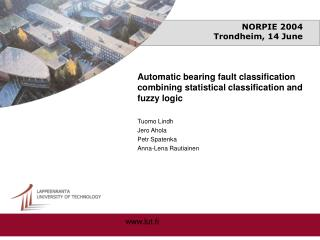# NORPIE 2004 Trondheim, 14 June - PowerPoint PPT PresentationDownload PresentationNORPIE 2004 Trondheim, 14 June

NORPIE 2004 Trondheim, 14 JuneDownload Presentation## NORPIE 2004 Trondheim, 14 June

- - - - - - - - - - - - - - - - - - - - - - - - - - - E N D - - - - - - - - - - - - - - - - - - - - - - - - - - -
##### Presentation Transcript

1. NORPIE 2004Trondheim, 14 June • Automatic bearing fault classification combining statistical classification and fuzzy logic • Tuomo Lindh • Jero Ahola • Petr Spatenka • Anna-Lena Rautiainen • www.lut.fi

2. Introduction to fault classification Neural networks, unsupervised Kohonen’s maps, supervised BP Statistical classification, Mahalanobis distance, Support vector Machines Hidden Markov chains, Fuzzy logic Adaptive, model based methods and many others

3. Why probabilistic and deterministic ? • Statistical pattern classification • search patterns of features • compare with known prototypes of faults • Deterministic calculation, rules • calculates the magnitudes • estimates the risk of fault classification • calculates the quality of features • avoid known pit falls (classification method, behaviour of machines etc.)

4. Appearance of cyclic bearing faults in motor frame vibration Wang (1998), Lindh (2002)

5. Statistical classification Minimum distance classifier

6. Fuzzy logic, the fault size estimation

7. Fuzzy logic, the probability of the fault uses the results of the statistical classification as input

8. Fault classifier

9. Results

10. Results Classification results using 16- dimensional feature space covering all four fault types. The bold cases are selected with the minimum distance classifier.

11. Results Classification results using four dimensional feature space. The distance between test vector and any fault prototypes are calculated separately. The bold cases are selected with the minimum distance classifier.

12. Results Classification results using simple fuzzy logic for the determination of fault degree

13. Results Classification results using fuzzy logic that estimates the fault degree as well as the probability of the faults. Action can be formed with fuzzy logic or multiplication of the fields of table of previous slide with the probabilities of this table. The actions are selected with trigger levels.

14. Conclusions • The method where the Mahalanobis distance based statistical classification results were used as one inputin fuzzy logic was introduced. • This input and other qualitative features of the spectrum were taken into account in the fuzzy logic which evaluated the probability of a certain fault. The other fuzzy logic evaluated the degree of certain fault and by combining the outputs of these logics, the final suggestion of the state and required action were given.

15. What?